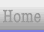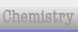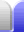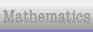# Electrical Units

### Table of units

 Quantity Common Unit Formula Symbol Current A, mA (milli-amp, milli-amp-hour) I Capacity Ah, mAh (amp-hour, milli-amp-hour) Voltage (potential difference) V (volt) V Resistance Ω (ohm) R Power W, mW (watt, milliwatt) P Energy Wh, mWh, J (watt-hour, milliwatt-hour, joule) E Intensity cd, mcd (candela, millicandela)

### Current

Current is measured in amps (actually amperes), symbol A (capital). One thousandth of an amp is a milliamp, symbol mA. It is named after André-Marie Ampère (person's name, gets a capital initial).

The capitalisation of mA is important. A million amps is a mega-amp, MA, and is really big. According to Wikipedia "an average bolt of negative lightning carries an electric current of 30 kiloamperes (kA) ... Large bolts of lightning can carry up to 120 kA". Meanwhile, ma is milliannums, or thousandths of a year (about a third of a day).

If used in a formula the symbol for current is I.

I = V / R

I = P / V

### Capacity

Capacity is how much current a battery can supply for how long. Capacity is measured in amp-hours, symbol Ah, or in milliamp-hours, symbol mAh. Note the capitalisation.

Voltage does not affect capacity, so several batteries placed in series have the same capacity as just one battery.

### Voltage

Electric potential is measured in volts (lower case initial unless at the start of a sentence), named after Alessandro Volta (person's name, gets a capital initial).

The symbol for volts is V (capital), both for the unit and when used in formulae.

V = I * R

V = P / I

### Resistance

Resistance is measured is ohms (lower case initial unless at the start of a sentence), named after Georg Simon Ohm (person's name, gets a capital initial).

The symbol for the ohms unit is Ω (the Greek letter capital omega). If used in a formula the symbol for resistance is R.

R = V / I

R = P / I2

### Power

Power is measured in watts (lowercase initial unless at the start of a sentence), named after James Watt (person's name, gets a capital initial).

The symbol for watts is W (capital), not to be confused with W, the chemical symbol for tungsten which is what incandescent light bulb filaments are made from. If used in a formula the symbol for power is P.

P = I * V

P = I2 * R

### Energy

The energy in a battery is found by multiplying power by time and is measured in watt-hours, symbol Wh, or in milliwatt-hours, symbol mWh.

Heat energy is measured in joules.

E = MC2

### 5 mm LED intensity

The intensity of 5 mm LEDs is measured with millicandela, symbol mcd (all lowercase). Most 5 mm LEDs have a domed top, which helps focus the light. Better focused LEDs, with a narrower beam, will have a higher intensity for the same total amount of light. 5 mm LEDs typically have ratings of several thousand millicandela, so candela (cd) would be a more wieldy and sensible unit... but that's not the way it seems to be done.Home Astronomy Chemistry Electronics & Computers Mathematics Physics Field Trips Turn on javascript for email link